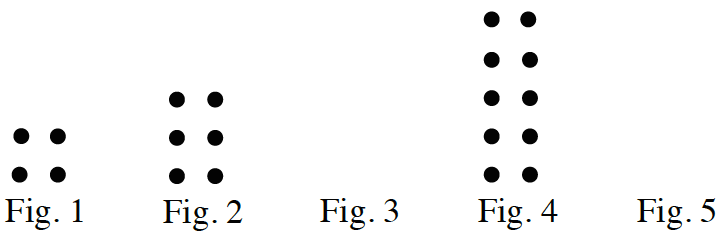### Home > CC1MN > Chapter 3 Unit 3A > Lesson CC1: 3.1.1 > Problem3-20

3-20.

Study the dot pattern below. 3-20 HW eTool (CPM). Homework Help ✎1. Draw the 3rd and 5th figures.

Can you see a pattern in the completed figures? Use this pattern to complete figures 3 and 5.

2. How many dots will there be in the 50th figure?

The number of dots can be found by multiplying the figure number by $2$ and then adding $2$. Do this with the figure number equal to $50$.

$102$ dots

3. Is there a figure that will have exactly $38$ dots? If so, which figure is it?

• Do the same process as above, but in reverse. Subtract $2$ and then divide by $2$. If you get a whole number, that is the figure number.

Use the eTool below to create the patterns for Fig. 3 and Fig. 5.
Click the link at right for the full version of the eTool: 3-20 HW eTool